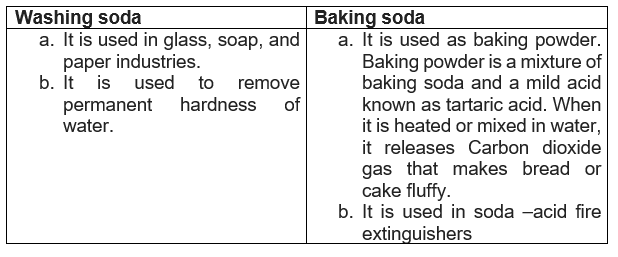# Acid Base and salts class 10 Intext and NCERT Solutions

## Intext NCERT Solutions

Page 18
Question 1
You have been provide with three taste tubes. One of them contains distilled water and the other two contains an acidic solution and a basic solution, respectively. If you are given only red litmus paper, how will you identify the contents of each tube?
We know from the chapter concept that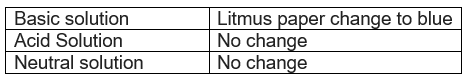Thus, basic solution can be easily identified.
When acid and a base react with each other. They neutralize each other to form salt and water.
So following steps can be taken to distinguish the test tubes with the help of litmus paper
Step 1: Let us mark the three taste tubes as P, Q, and R respectively
Step 2 A drop of solution in taste tubeP is put on the red litmus paper. Same is repeated with solutionQ and R. If either of them change colour to blue, then it is basic. Therefore, out of three, one is eliminated.
Step 3 Out of remaining two, anyone can be acidic or neutral. Now a drop of basic solution is mixed with a drop of each of the remaining two solutions separately and then a drop of each solution is put on the red litmus paper.
If the colour of red litmus turns blue, then that solution is neutral and if there is no change in colour, then that solution is acidic.
This is because acidic and basic solutions neutralize each other. Hence , we can distinguish between the three types of solutions.

Page 22
Question 2
Why should curd and sour substances not be kept in brass and copper vessels?
Curd and other sour substances contain acids. Therefore , when they are kept in copper and brass vessels, the metal reacts with the acid to liberate hydrogen gas and harmful products, thereby spoiling the food.

Question 3
which gas is usually liberated when an acid reacts with a metal? Illustrate with an example . How will you test for the presence of this gas?
Hydrogen gas is usually liberated when an acid reacts with a metal.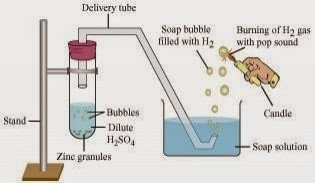Example
Procedure:
Step 1: Take few pieces of zinc granules and add 5 ml of dilute H2 SO 4
Step 2: Shake it and pass gas produced into a soap solution. The bubbles are formed in the soap solution. These soap bubbles contain hydrogen gas.
Zn + dil. H2 SO 4 --àZnSO 4 + H 2
Test for hydrogen gas: The evolved hydrogen gas can be tested by bringing a burning candle near the soap bubbles. Hydrogen gas burns with a pop sound.

Question 4
Metal compound A reacts with dilute hydrochloric acid to produce effervescence. The gas evolved extinguishes a burning candle. Write a balanced chemical equation for the reaction if one of the compounds formed is calcium chloride.
Metal compound A should be compounds of Ca as a calcium salt is formed in the product. We know that CO 2 extinguishes a burning candle. Also , since carbon dioxide is liberated, therefore, it must be a carbonate. Hence, it is calcium carbonate.
CaCO 3(S) + 2HCL(aq) -> CaCL 2(S) + CO 2(g) + H 2O(I)
Hence metal compound A is calcium carbonate.

Page 25
Question 5
Why do HCL, HNO 3, etc., show acidic characters in aqueous solutions while solution of compounds like alcohol and glucose do not show acidic character?
HCL and HNO 3 dissociate in the presence of water to form hydrogen or hydronium ions.
Although aqueous solutions of glucose and alcohol contain hydrogen , these cannot dissociate in water to form hydrogen or hydronium ions. Hence they do not show acidic character.

Question 6
Why does an aqueous solution of acid conduct electricity?
Acid dissociate in aqueous solutions to form ions. These ions are responsible for conduction of electricity.

Question 7
Why does dry HCL gas not change the colour of the dry litmus paper?
Dry HCL gas does not change the colour of the dry litmus paper because it does not contain hydrogen or hydronium ions.

Question 8
While diluting an acid , why is it recommended that the acid should be added to water and not water to the acid?
As the reaction between an acid and water is exothermic reaction, It is recommended that acid should be added to water and not water to the acid because the process of dissolving an acid in water is exothermic . If water is added to acid , since large amount of acid is present, a large amount of heat is generated, the mixture splashes out and causes burns.

Question 9
How is the concentration of hydronium ions (H 3O+) affected when a solution of an acid is diluted?
When an acid is diluted, the concentration of hydronium ions (H 3O +) per unit volume decreases. This means that the strength of the acid decreases.

Question 10
How is the concentration of hydroxide ions (OH -) affected when excess base is dissolved in a solution of sodium hydroxide?
the concentration of hydroxide ions (OH -) would increase when excess base is dissolved in a solution hydroxide.

Page 28
Question 11
You have two solutions A and B . The pH of solution A is 6 and pH of solution B is 8. Which solution has more hydrogen ion concentration ? Which of this acidic and which one is basic?
We know from the chapter concept that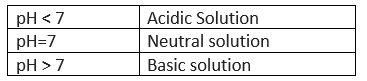A pH value of less than 7 indicates an acidic solution, while greater than 7 indicates a basic solution. Therefore, the solution with pH = 6 is acidic and has more hydrogen ion concentration then the solution pH = 8 which is basic.

Question 12
What effect does the concentration of H +(aq) ions have on the nature of the solution?
The concentration of H + ions is directly proportional to the acidic of a solution.
If the concentration of H+ ions is increased, the solution becomes more acidic. If the concentration of H+ ions decreased, the solution becomes less acidic or more basic.

Question 13
Do basic solution also have H + (aq) ions? If yes then why are these basic?
Yes, a basic solution also has H + (aq) ions. However their concentration is less as compared to the concentration of OH - (aq) ions that makes the solution basic.

Question 14
Under what soil condition do you think a farmer would treat the soil of his field with quick lime (calcium oxide) or slaked lime (calcium hydroxide) or chalk (calcium carbonate)?
As calcium oxide , calcium hydroxide and calcium carbonate are basic in nature. So, when an acid is base react , a neutralization reaction takes place.So If the soil is acidic and improper for cultivation, then to neutralize the acidity of the soil, the farmer would treat the soil with quick lime or slaked lime or chalk.

Page 33
Question 15
What is common name of compound CaOCL 2?
Bleaching powder .

Question 16
Name the substance which on treatment with chlorine yields bleaching powder?
Calcium hydroxide [Ca(OH)2]

Question 17
Name sodium compound which is used for softening hard water?
Washing soda (Na2 CO 3.10H2O) is used for softening hard water.

Question 18
What will happen if a solution of sodium hydrocarbonate is heated?
When a solution of sodium hydrocarbon ate is heated, sodium carbonate and water are formed with the evolution of carbon dioxide gas.
2NaHCO3 -> Na 2CO 3 +H 2O + CO 2
NaHCO 3 : sodium hydrocarbonate Na2CO3 : Sodium Carbonate

Question 19
Write an equation to show the reaction between Plaster of Paris and water?
The chemical equation for the reaction of Plaster of Paris and water can be represented as:
CaSO 4.(1/2) H 2O +1 ½ H 2O ->CaSO 4.2H 2O
Plaster of pares -> CaSO 4.(1/2) H 2O Gypsum ->CaSO 4.2H 2O

## NCERT Exercise Solutions

Question 20
A solution turns red litmus blue, its pH is likely to be
(a) 1
(b) 4
(c) 5
(d) 10
Bases turns red litmus blue and acids turn litmus red . Basic solution has a pH value more than 7. Since the solution turns red litmus blue, its pH is likely to be 10
Question 21
A solution reacts with crushed egg-shells to give a gas that turns lime water milky. The solution contains
(a) NaCL
(b) HCL
(c) LicL
(d) KCl

Question 22
10 mL of a solution of NaOH is found to be completely neutralized by 8 mL of a given solution of HCL. If we take 20mL of the same solution of NaOH, the amount of HCL solution (the same solution as before) required to neutralize it will be
(a) 4mL
(b) 8mL
(c) 12mL
(d) 16mL
(d) 16mL

Question 23
Which one of the following types of medicines is used for treating indigestion?
(a) Antibiotic
(b) Analgesic
(c) Antacid
(d) Antiseptic
Antacid

Question 24
Write word equation and then balanced equations for the reaction taking place when-
(a) Dilute sulphuric acid reacts with zinc granules.
(b) Dilute hydrochloric acid reacts with magnesium ribbon.
(c) Dilute sulphuric acid reacts with aluminum powder.
(d) Dilute hydrochloric acid reacts with iron filings.
How to solve these type of Question
• First convert the word equation into skeletal equation
• Start by finding out how many atoms of each type are on each side of the equation
• You can make a little table listing the numbers of each atom for the left hand side and for the right hand side
• Balance the different Atoms on both side of equation by multiply the chemical species on the side which doesn't have enough atoms of that type by the number required to bring it up to the same as the other side.
(a) Sulphuric acid + Zinc --> Zinc sulphate + Hydrogen
H 2SO 4(aq) + Zn(s) -- > ZnSO 4(aq) + H 2(g)
(b) Hydrochloric acid + Magnesium ---> Magnesium chloride + Hydrogen
2HCL(aq) + Mg(s) --> MgCl 2(aq) + H 2(g)
(c) Sulphuric acid + Aluminium --> Aluminum sulphate + Hydrogen
3H 2SO 4(aq) + 2Al(s) ---> Al 2(SO 4) 3 (aq) +3H 2(g)
(d) Hydrochloric acid + iron --> Ferric chloride + Hydrogen
6HCl (aq) + 2Fe(s) ---> 2FeCl 3(aq) + 3H 2(g)

Question 25
Compound such as alcohols and glucose also contain hydrogen but are not categorized as acids. Describe an activity to prove it.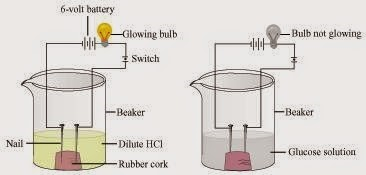Procedure:
Step 1: Two nails are fitted in a cork and are kept it in a 100mL beaker.
Step 2: The nails are then connected to the two terminals of a 6-volt battery through a bulb and switch.
Step 3: Some dilute HCl is poured in the beaker and current is switched on.
Step 4: The same experiment is then performed with glucose solution and alcohol solution.
Observations: It will be observed that the bulb glows in the HCl solution and does not glow in the glucose solution.
HCl dissociate into H + (aq) and Cl -(aq) ions. These ions conduct electricity in the solution which results in the glowing of the bulb. On the other hand, the glucose solution which does dissociate into ions. Therefore, it does not conduct electricity.
Conclusion: From this activity, it can be concluded that all acid contain hydrogen but not all compounds containing hydrogen are acids.
That is why , though alcohol and glucose contain hydrogen , they are not categorized as acids.

Question 26
Why does distilled water not conduct electricity , whereas rain water does?
Distilled water is a pure form of water and is devoid of any ionic species. Therefore , it does not conduct electricity . Rain water , being an impure form of water , contains many ionic species such as acid and therefore, it conducts electricity.

Question 27
Why do acids not show acidic behavior in the absence of water ?
Acids do not show acidic behavior in the absence of water because there are no hydrogen ions available for conduction of electricity. Acids dissociate in the presence of water to give free hydrogen ions. It is the hydrogen ions that are responsible for the acidic behavior.

Question 28
Five solutions A,B,C,D and E when tested with universal indicator showed pH as 4,1,11,7 and 9, respectively. Which solution is
(a) neutral?
(b) strongly alkaline?
(c) strongly acidic?
(d) weakly acidic?
(e) weakly alkaline?
Arrange the pH in increasing order of the concentration of hydrogen ions
We know from the chapter concept that(a) Neutral →Solution D with pH 7
(b) Strongly alkaline →Solution C with pH 11
(c) Strongly acidic →Solution B with pH 1
(d) Weakly acidic →Solution A with pH 4
(e) Weakly alkaline →Solution E with pH 9
Arrangement in increasing order of concentration of hydrogen ions 11<9<7<4<1

Question 29
Equal lengths of magnesium ribbons are taken in the test tubes A and B. Hydrochloric acid (HCl) is added to test tube A, while acetic acid (CH 3COOH) is added to test tube B. In which test tube will the fizzing occur more vigorously and why?
The fizzing will occur strongly on the test tube A, in which hydrochloric acid (HCl) is added. This is because HCl is a stronger acid then CH 3COOH and therefore produces hydrogen gas at a faster speed due to which fizzing occurs.

Question 30
Fresh milk has a pH of 6. How do you think the pH will change as it turns into curd? Explain your answer.
We know from the chapter concept thatThe pH of milk is 6. As it changes to curd , the pH will reduce because curd is acidic in nature. The acid present in it decrease the pH.

Question 31
A milkman adds a very small amount of baking soda to fresh milk.
(a) Why does he shift the pH of the milk from 6 to slightly alkaline?
(b) Why does this milk take a long time to set as curd?
(a) The milkman shift the pH of the fresh milk from 6 to slightly alkaline because in alkaline condition, milk does not set as curd easily.
(b) Since this milk is slightly basic than usual milk , acids produced to set the curd are neutralized by the base. Therefore , it takes a longer time for the curd to set.

Question 32
Plaster of Paris should be stored in moisture –proof container. Explain why?
Plaster of Paris should be store in moisture – proof container because Plaster of Paris, a powdery mass , absorbs water (moisture) to form a hard solid known as gypsum.
CaSO 4.1/2 + 1 1/2H 2O --> CaSO 4.2H 2O

Question 33
What is neutralization reaction? Give two examples.
Neutralization reaction: A reaction in which an acid and base react with each other to give a salt and water is termed as neutralization reaction. In this reaction, energy is evolved in the form of heat.
For example: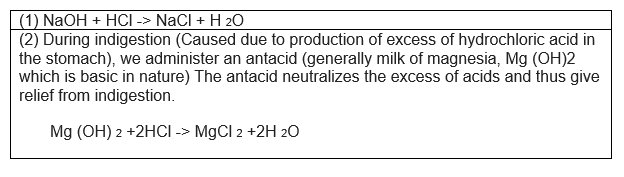Question 34
Give two important uses of washing soda and baking soda?
Two important used of washing soda and baking soda is as follows: